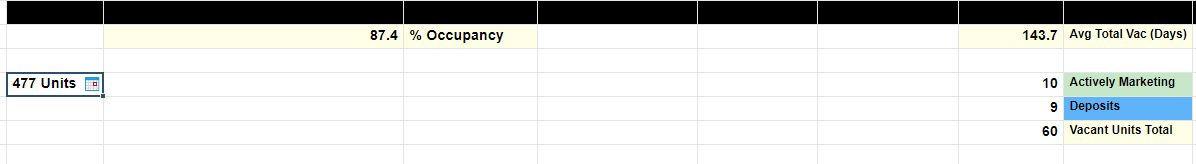# Inserting a Formula into an existing Equation

I have a simple math equation in one of my cells, and in place of one of the numbers I would like to reference a count of cells with dates in them in a certain column.

My equation is: =100 - (((60 / 477) * 100))  (this returns a number in a percentage format) and I would like to replace the number "60" with this: =COUNT([Today Date]2:[Today Date]61)

I can't seem to get it to taken maybe my parenthesis placement is wrong?

I would also be fine replacing the 60 with a reference to the cell with the equation in it if that is easier, so it just pulls whatever number results from my =COUNT formula. I could not get that to take either.

I attached a screeshot, I am basically trying to get a total vacant unit count by counting the rows, and then use that number to get a total occupancy percentage

Thank you anyone who can help!• =100 - (count([Today Date]2:[Today Date]61) / 477) * 100))

Should do the trick. You will get a total count of everything in row 2 - 61.

• I did attempt that, and I get the #UNPARSEABLE error, unfortunately. Thank you for trying to help though!

• Try this: =100 - (COUNT([Today Date]2:[Today Date]61) / 477) * 100

There were some issues with the parenthesis.

• My hero! This did the trick, thanks much!

• Glad that I got that working for you!

## Help Article Resources

Want to practice working with formulas directly in Smartsheet?

Check out the Formula Handbook template!